# Superalgebra

A-graded algebra over a field(see Graded algebra), i.e. a super-spaceoverendowed with an even linear mapping. A superalgebra is said to be commutative (graded-commutative or supercommutative) ifhere,is a parity, i.e. a-grading.

The definition of a superalgebra can be generalized to include the case where the domain of scalars is an arbitrary commutative associative superalgebra.

Examples of associative superalgebras overare: the algebraof matrices of the form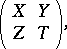where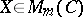,, endowed with the natural-grading (cf. Super-space); the tensor algebraof a-graded moduleover; the symmetric algebra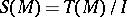of a module, whereis the ideal generated by the elements of the form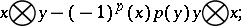and the exterior algebra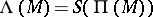of a module(the latter two superalgebras are commutative).

A superalgebra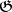with a multiplication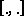is called a Lie superalgebra if for all,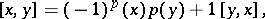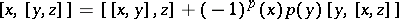(andif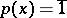and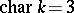). In particular, there are no Lie superalgebras in characteristic, only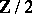-graded Lie algebras.

Examples. Any associative superalgebra endowed with commutation (the supercommutator difference)as the bracket operation; the algebraof derivations of an arbitrary superalgebra(i.e. of linear transformations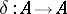for which) with the operation of commutation. For any Lie superalgebrathere is an associative universal enveloping superalgebra, and the straightforward generalization of the Birkhoff–Witt theorem holds.

The classification of finite-dimensional simple Lie superalgebras over the fieldis known (see , ). They are divided into Lie superalgebras of classical type (characterized by the fact that the Lie algebra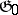is reductive) and Lie superalgebras of Cartan type. The Lie superalgebras of classical type are exhausted by the following series of matrix algebras: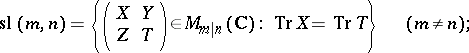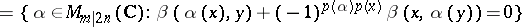for an even symmetric non-degenerate bilinear form;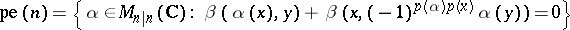for an odd symmetric non-degenerate bilinear form;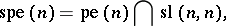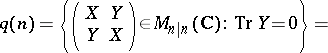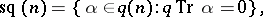where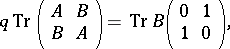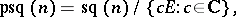and certain exceptional algebras (of dimensions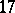,and). The superalgebras of Cartan type are the algebra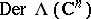and its supersubalgebras, analogues to the simple Lie graded algebras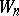,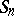,(cf. Lie algebra, graded).

The classification of real structures of simple Lie superalgebras and a description of semi-simple Lie superalgebras in terms of simple ones are also known.

The theory of linear representations of Lie superalgebras is essentially more complex than for Lie algebras in that representations of simple Lie superalgebras, as a rule, are not completely reducible, while irreducible representations of solvable Lie superalgebras need not be one-dimensional. A classification exists of the irreducible representations of simple finite-dimensional Lie superalgebras overin terms of the highest weights (see , ), and an explicit description is known of the finite-dimensional representations, as well as for the character formula for certain series of these algebras .